# Gases

Also found in: Dictionary, Thesaurus, Medical, Legal, Acronyms, Idioms.
The following article is from The Great Soviet Encyclopedia (1979). It might be outdated or ideologically biased.

## Gases

the state of aggregation of a substance whose particles are not bound or are only weakly bound by the forces of interaction and whose particles move freely, entirely filling the available volume.

Substances in a gaseous state are common in nature. Gases form the atmosphere of the earth, they are contained in solid terrestrial rock and are dissolved in ocean, sea, and river water. The sun, the stars, and interstellar clouds are composed of neutral or ionized gases (plasma). Gases are usually encountered in nature as mixtures of chemically individual gases.

Gases have a number of characteristic features. They completely fill the vessel that contains them and take the shape of that vessel. In contrast to solids and liquids, the volume of a gas depends largely on pressure and temperature. Under normal conditions (0°-100° C) the coefficient of the volumetric expansion of gases is two orders higher than that of liquids; on the average it is 0.003663 deg-1. Physical properties of the most common gases are shown in Table 1.

Table 1. Physical properties off gases
Nitrogen (N2)Argon (Ar)Hydrogen (H2)AirOxygen (O2)Carbon dioxide (CO2)
1 1 atm = 9.81 × 104 N/m2
Mass of 1 mole (g) ................28.0239.942.01628.9632.0044.00
Density at 0°C and 1 atm1 (kg/m3) ................1.25061.78390.08991.29281.42901.976
Heat capacity at constant volume cυ and 0° C [kJ/(mole.deg)] ................20.8512.4820.3520.8120.8930.62
(55 ° C)
Speed of sound at 0° C (m/sec) ................333.63191,286331.5314.8260.3
Viscosity η atO°C (η-105 N.sec/m2) ................16.621.28.417.119.213.8
Heat conductivity λ at 0° C [(λ-102 J/(m.sec.° K)] ................2.431.6216.842.412.441.45
Dielectric constant є at 0° C and 1 atm1 ................1.0005881.0005361.0002721.0005901.0005311.000988
Specific magnetic susceptibility k at 20° C (k.106 per g) ................-0.43-0.49-1.99+107.8-0.48

Any substance can be transformed into a gaseous state by the proper combination of pressure and temperature. Therefore, the zone of possible existence of the gaseous state may be conveniently shown on a graph by the variables p (pressure) and T (temperature): see the (p,T) diagram in Figure 1. At temperatures below the critical temperature Tc this zone is bounded by the curves of sublimation (I) and vaporization (II). This means that at any pressure below the critical pressure Pc there is a temperature T, determined by the curve of sublimation or vaporization, above which the substance turns into a gas. Along curve I (below the triple point Tp) the gas remains in equilibrium with the solid matter (solid phase); along curve II (between the triple point and the critical point C) the gas is in equilibrium with the liquid phase. Gas in these conditions is usually called the vapor of a substance.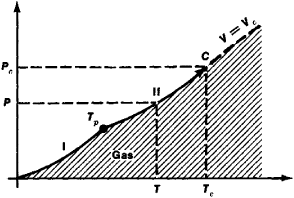Figure 1. (p,T) diagram of the state of a substance. The zone of the gaseous state is shaded. The curves of sublimation (I) and vaporization (II) mark its lower temperature and pressure boundaries. Tp is the triple point; C is the critical point. The dotted line indicates the critical isochor of the substance.

At temperatures below Tc gases may condense, that is, they may pass into the other states of aggregation (solid or liquid). It should be noted that the phase transition of gases into liquids or solids takes place stepwise: a slight change in pressure leads ultimately to changes in a number of properties of a substance, such as density, enthalpy, and heat capacity. The processes of gas condensation, especially liquefaction, are important for industry.

At T > Tc the boundary of the gaseous region is arbitrary, since phase transitions do not occur at these temperatures. In a number of cases the critical isochor of a substance (the curve of constant density or specific volume; see Figure 1), in the immediate vicinity of which the properties of the substance change especially quickly, although not stepwise, is taken as the arbitrary boundary between gases and liquids at supercritical temperatures and pressures.

Since the region of the gaseous state of a substance is very large, the properties of a gas may change over a wide range upon changes in temperature and pressure. Under normal conditions (at 0° C and atmospheric pressure) gas density is about 1,000 times less than the density of the same substance in a solid or liquid state. At room temperature and a pressure 1017 times lower than atmospheric pressure (the limit of modern vacuum technology) the density of gases is about 10-20 g/cm3. Under conditions of outer space, gas pressure can be ten orders lower (~10-30 g/cm3).

On the other hand, at high pressures a substance that may be considered a gas at supercritical temperatures has enormous density (for example, in the center of some stars it is ~109 g/cm3). Other properties of gases, such as heat conductivity and viscosity, may vary widely depending on the conditions.

Kinetic molecular theory of gases. According to the kinetic molecular theory, gases are considered to be the aggregate of weakly interacting particles (molecules and atoms) that are constantly in chaotic (thermal) motion. Based on such simple representations, kinetic theory can explain the basic physical properties of gases, especially the properties of rarefied gases.

Gases that are sufficiently rarefied have an average distance between molecules that is much greater than the effective radius of intermolecular forces. Thus, for example, under normal conditions 1 cm3 of a gas contains ~1019 molecules, and the average distance between them is ~10-6 cm, or ~100 angstroms (Å), whereas at distances greater than 5-10 Å, molecular interaction is not significant. Under such conditions molecules interact only when they approach one another to the distance at which the intermolecular forces are effective. Such an approach is customarily called a collision of molecules. The effective radius of intermolecular forces in the example given is 10-20 times smaller than the average distance between molecules, so that the total volume in which these forces are significant (the “intrinsic volume” of all the molecules) is 10-3 to 10-4 of the total volume of the gas. This makes it possible to consider the volume of the gas molecules themselves to be negligibly small under normal conditions and to regard the molecules as material points. A gas whose molecules are considered to be noninteracting material points is called ideal. Under conditions of thermal equilibrium the molecules of an ideal gas move in all directions with equal probability at speeds distributed according to the Maxwell distribution. This distribution for nitrogen at 20° C and 500° C is shown graphically in Figure 2. The graph reveals that the speed values for the overwhelming majority of molecules are close to each other; the maximum of the curve corresponds to the most probable speed at a given temperature. However, there is a certain portion of the molecules with low and very high speeds. Using the Maxwell distribution it impossible to determine the so-called mean square speed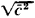of the molecules, which is related to the temperature T of the gas by the equation

c-2=3kT/m

where k is the Boltzmann constant and m is the mass of a molecule. Equation (1) makes it possible to establish the connection between the average kinetic energy of one molecule and the temperature of the gas: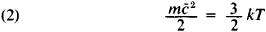This relationship is often considered to be the kinetic molecular explanation of temperature—that temperature is the measure of the kinetic energy of a molecule.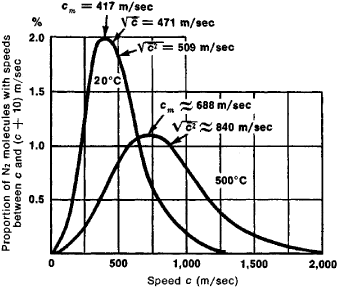Figure 2.

Note to Figure: Maxwell distribution for nitrogen molecules at temperatures of 20° and 500°C. The proportion of molecules (in percent) having speeds between c and (c + 10) m/sec is plotted on the y-axis; cm is the most probable speed that the largest number of molecules will have at a given temperature; c is the arithmetic mean speed of the molecules;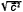is the root mean square speed.

Since the molecules of an ideal gas have only kinetic energy, the internal energy of such a gas does not depend upon the volume it occupies (Joule’s law).

Kinetic molecular theory considers gas pressure on the walls of a vessel containing the gas to be the effect of molecules striking the walls averaged over the surface and over time. The pressure p is quantitatively determined by the momentum transferred by molecules per unit of time to a unit of area of the wall:

(3) p=1/3nmc-2

where n is the number of molecules per unit volume. Equations (2) and (3) make it possible to write an equation of the state of an ideal gas in the form

(4) p = nkT

Equation (4), which is written for one mole of a gas, or N = 6.022 × 1023 molecules, is called Clapeyron’s equation:

(5) pv = RT

where R = kN is the universal gas constant and v is the volume per mole. Clapeyron’s equation generalizes the empirical Boyle-Mariotte and Gay-Lussac gas laws. It also follows from equation (5) that at the same pressure and temperature ideal gases taken in a quantity of 1 mole have equal volumes and that any such gas has the same number of molecules as any other such gas per unit volume (Avogadro’s law). Under conditions of thermal equilibrium, gas temperature and pressure are equal throughout its entire volume, the molecules move about chaotically, and there are no regular streams. The formation of temperature or pressure drops (gradients) leads to the disruption of equilibrium, and energy, mass, or other physical quantities shift in the direction of the gradient.

The kinetic properties of gases—heat conductivity, diffusion, and viscosity—are seen in kinetic molecular theory from the same point of view: diffusion is considered to be a transfer of mass by the molecules; heat conduction, a transfer of energy by the molecules; and viscosity, a transfer of momentum by the molecules. The model of an ideal gas is unsuitable for analysis of the transfer phenomena because in these processes the collisions and the “size” of molecules play the essential part; the collisions provide the occasion for the transfer of any quantity (such as energy), and the “size” of the molecules influences the frequency of collisions. Therefore, in the simplest case, transfer phenomena are considered for a rarefied gas, whose molecules are initially viewed as elastic spheres with a definite diameter σ the spheres interact only at the moment of collision. In this approximation the diameter of a molecule is in the following simple relation with its mean free path length :

(6) = 1/πσ2.n

The magnitude of significantly influences the transfer processes in a rarefied gas. In particular, if the characteristic dimension of the volume occupied by a gas is greater than , then the heat conductivity and viscosity of the gas do not depend on its pressure. On the other hand, when is greater than the characteristic dimension, then the heat conductivity and viscosity tend to drop (which also means a decrease in the number of collisions) if the pressure decreases. This phenomenon underlies the thermal insulation properties of vessels with double walls between which the air has been evacuated.

When the transfer phenomena in rarefied gases are analyzed in more accurate molecular theory, the interaction of molecules is taken into account at all distances between them. The type of interaction is determined by the so-called interaction potential. Accurate examination of the dynamics of paired interaction (collisions) has led to the inclusion of so-called collision integrals in formulas for calculating the transport coefficients. These collision integrals are functions of the given temperature T* = kT/є. This temperature characterizes the ratio of the molecules’ kinetic energy (~kT) to their potential energy (є is the depth of the potential well at a given potential of interaction). Collision integrals take into account the fact that, depending on the kinetic energy (and hence the temperature of the gas), colliding molecules may approach to various distances, as if they were changing their effective size.

Properties of real gases. When the density increases, the properties of a gas change, and it ceases to be ideal. The equation of state (5) is found to be inapplicable, since the mean distances between molecules of the gas become comparable to the radius of molecular interaction. To describe the thermodynamic properties of nonideal or, as they are more often called, real gases, various equations of state are used; these equations have a more or less strict theoretical foundation. The van der Waals equation is the simplest example of an equation that qualitatively correctly describes the basic differences between real gas and ideal gas. On the one hand it takes into account the existence of forces of attraction between molecules that leads to a decrease in gas pressure; on the other hand, the existence of ferees of repulsion, which prevent infinite compression of gases.

The virial equation of state is the best grounded theoretically, at least for states far from the critical point: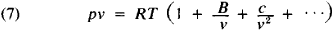The values of the virial coefficients B, C, … are determined by the collisions of molecules: binary (B), triple (C), and of a higher order for the subsequent coefficients. It is essential that virial coefficients are functions only of temperature.

In a low-density gas, binary collisions of molecules are most likely; that is, for a gas that is expanding (7) it is possible to omit all the terms after the term with the B coefficient. In accordance with the temperature change of B, B becomes zero at the so-called Boyle temperature TB, and a moderately dense gas behaves like an ideal gas, that is, it follows equation (5). From the point of view of physics, this means that at TB intermolecular forces of attraction and repulsion virtually cancel each other out. The existence of molecular interaction influences to a certain degree all the properties of a real gas. The internal energy of a real gas is found to be dependent on its volume—that is, on the distance between molecules— because the potential energy of molecules is determined by their distance from one another.

The change in temperature of a real gas when it flows at a low constant speed through a porous partition (a process called throttling) also depends on molecular interaction. The Joule-Thomson coefficient serves as a measure of the temperature change of a gas during throttling. Depending on the circumstances, this coefficient may be either positive (gas cooling), negative (gas heating), or zero (at the so-called inversion temperature). The effect of gas cooling during throttling is widely used in industry as one of the methods of liquefaction of gases.

The internal structure of gas molecules has a weak influence on their thermodynamic properties (pressure, temperature, density, and the relations between them). Only molecular mass is essential for these properties in the first approximation. However, the caloric properties of a gas (heat capacity, entropy, and so on), as well as its electrical and magnetic properties, depend essentially on the structure of the molecules. For example, in order to calculate (in a first approximation) the heat capacity of a gas at constant volume cυ, it is necessary to know the number of internal degrees of freedom iint of a molecule (that is, the number of its possible internal movements). According to the equipartition law of classical statistical physics, the energy of each degree of freedom of a gas molecule (translational, oscillatory, rotational) is equal to 1/2kT. Hence the heat capacity of 1 mole is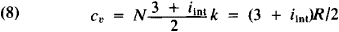To calculate exactly the caloric properties of gases, the energy levels of its molecules must be known; this can usually be learned by analysis of the spectra of the gases. The caloric properties of a large number of substances in the state of an ideal gas have been calculated with high precision, and their values are given in the form of tables up to temperatures of 10,000°-22,000° C.

The electrical properties of gases depend chiefly on the possibility of ionization of their molecules or atoms, that is, on the occurrence in the gases of electrically charged particles (ions and electrons). If there are no charged particles, gases are good dielectrics. As the charge concentration increases, the electrical conductivity of gases increases.

At temperatures of several thousand degrees and above, all gases become partially ionized and turn into plasma. If the charge concentration in plasma is small, then its properties are only slightly different from the properties of normal gases.

According to their magnetic properties, gases may be categorized as diamagnetic (for example, the inert gases and H2, N2, CO2, and H2O) or paramagnetic (for example, O2). Diamagnetic gases are gases whose molecules do not have a constant magnetic moment and acquire it only under the influence of an external field. Gases whose molecules have a constant magnetic moment behave like paramagnetics in an external magnetic field.

The molecular interaction and the internal structure of the molecules must be considered when solving many problems in gas physics—for example, when investigating the influence of the rarefied gases of the upper strata of the atmosphere on the movement of rockets and artificial earth satellites.

In modern physics, more than one of the states of aggregation of matter is called gas. The aggregate of free electrons in metal (electron gas) and the phonons in liquid helium (phonon gas) are included among gases with special properties. Gases of elementary particles and quasi-particles with integral spin, the so-called bosons (for example, photons, pimesons, and phonons), are called a Bose gas. Its properties are examined in Bose-Einstein quantum statistics. The properties of gas particles with half-integral spin—fermions (for example, electrons, neutrons, neutrinos, conductivity holes) —are examined in Fermi-Dirac quantum statistics.

### REFERENCES

Kirillin, V. A., V. V. Sychev, and A. E. Sheindlin. Tekhnicheskaia termodinamika. Moscow, 1969.
Kikoin, I. K., and A. K. Kikoin. Molekuliarnaia fizika. Moscow, 1963.
Hirschfelder, J., C. Curtis, and R. Bird. Molekuliarnaia teoriia gazov i zhidkostei. Moscow, 1961. (Translated from English.)
Termodinamicheskie svoistva individual’nykh veshchestv: Spravochnik, 2nd ed., vols. 1-2. Edited by V. P. Glushko. Moscow, 1962.

E. E. SHPIL’REIN

References in periodicals archive ?
That's because most researchers studying those gases use such perfect crystals that electrons move ballistically, that is, they travel extraordinary distances--tens to hundreds of micrometers--before colliding with one another or with atoms in the material.
In Kilauea's runny lava, some trapped gases escape during its lengthy trip--12 kilometers (7 miles)--from its vent to the Pacific Ocean.
The pouring temperature also is critical in ensuring trapped gases are out of the casting.
In many instances, manufacturing processes use or generate synthetic chemicals that, pound for pound, absorb much more of the sun's infrared radiation than natural greenhouse gases, such as carbon dioxide, do.
Cooled gases pass through a large, low-pressure drop spark arrestor prior to entering the baghouse at 450F (232c).
Researchers instead focused on other ways to reduce carbon dioxide pollution, such as increasing energy efficiency and developing alternative power sources that emit little or no greenhouse gases.
Nitrogen has been shown to create a very wet dross, argon somewhat drier, with the driest dross created by halogen containing purge gases.
Almost all current binder systems produce significant amounts of gases during casting--it would be difficult to design a binder that did not do so.
Cars spew gases such as carbon monoxide into the air mostly during stop-and-go traffic, when they spend a lot of time idling.
A gas hole forms when the gas pressure, at any point, exceeds the head pressure and is influenced by the mold's ability to release the gases generated in the mold (permeability).
These behemoths also possess huge amounts of hydrogen and helium gases, which form their thick atmospheres.

Site: Follow: Share:
Open / Close